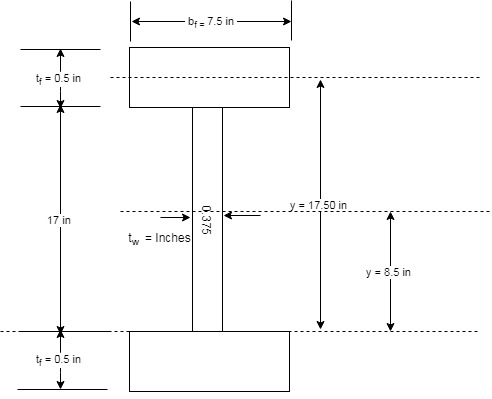# A flexural member is fabricated from two flange plates 1 2 × 7 1 2 and a web plate 3 8 × 17 . The yield stress of the steel is 50 ksi. a . Compute the plastic section modulus Z and the plastic moment M p with respect to the major principal axis. b . Compute the elastic section modulus S and the yield moment M y with respect to the major principal axis.### Steel Design (Activate Learning wi...

6th Edition
Segui + 1 other
Publisher: Cengage Learning
ISBN: 9781337094740### Steel Design (Activate Learning wi...

6th Edition
Segui + 1 other
Publisher: Cengage Learning
ISBN: 9781337094740

#### Solutions

Chapter 5, Problem 5.2.1P
Textbook Problem

## A flexural member is fabricated from two flange plates 1 2 × 7 1 2 and a web plate 3 8 × 17 . The yield stress of the steel is 50 ksi.a. Compute the plastic section modulus Z and the plastic moment M p with respect to the major principal axis.b. Compute the elastic section modulus S and the yield moment M y with respect to the major principal axis.

Expert Solution
To determine

(a)

Plastic section modulus Z and the plastic moment Mp with respect to the major principal axis.

The plastic section modulus Z=185.437in3 and the plastic moment Mp=772.65ft.kips with respect to the major principal axis.

### Explanation of Solution

Given:

A flexural member is fabricated from two flange plates 12×712 and a web plate 38×17. The stress of the steel is 50Ksi.

Concept used:

The section is a symmetrical section which implies that the plastic neutral axis of the given section is same as the neutral of the given section. Therefore, calculating the lever arm and the centroid of the upper half of the given section, we can find the plastic section modulus.

We have the following figure that will define the terms that we have been given as per the question.Calculation:

The following is the tabular measurement of every component required:

 Elements h (inches) b (inches) A  =h ×b  (inches)2 y  (inches) Ay  (inches)3 Web 38  =  0.375 172  =  8.5 8.5  ×  0.375  =  3.1875 8.50 27.09 Flange 12  = 0.5 712  = 7.5 0.5  ×  7.5 =  3.75 17.50 65.625 Sum ∑A = 6.94 ∑y =26.00 ∑Ay  = 92.72

Calculating the centroid of the top half as :

y¯=AyA

Substitute the values in the above equation.

y¯=Ay=92.72inA=6.94iny¯=13.36in.

Now, calculating the moment arm, we have the following formula :

a=2×y¯

Where, a is the moment arm of the section.

a=2×y¯a=2×13.36in.a=26.72in.

Now, the plastic section modulus can be calculated as follows:

Z=(A2)×a

Where, Z is plastic section modulus and A is area.

Z=(A2)×aZ=6.94in2×26.72in.Z=185.437in3.

Calculating the plastic moment as follows:

Mp=Fy×Z

Substitute the value of Fy and Z, we have

Mp=Fy×ZMp=50Ksi×185.437in3.Mp=9271.85in.kips.Mp=772.65ft.kips.

Conclusion:

Therefore, the plastic section modulus Z=185.437in3 and the plastic moment Mp=772.65ft.kips with respect to the major principal axis.

Expert Solution
To determine

(b)

Elastic section modulus, S and the yield moment, My of the section with respect to the major principal axis.

The elastic section modulus, S=272.284in3 and the yield moment of the section with respect to the major principal axis is My=1134.52ft.kips.

### Explanation of Solution

Given:

A flexural member is fabricated from two flange plates 12×712 and a web plate 38×17. The stress of the steel is 50Ksi.

Concept used:

The section is a symmetrical section which implies that the elastic neutral axis of the given section is coinciding with the neutral of the given section. Therefore, calculating the moment of inertia at the major axis using parallel axis theorem, we can find the elastic section modulus.

We have the following figure that will define the terms that we have been given as per the question.Calculation:

The following is the tabular measurement of every component required:

 Elements I ¯(inches)4 A  =h ×b  (inches)2 d  (inches) I=I¯+A×d2(inches)4 Web 153.53 17  × 38  =  6.375 0.00 153.53 Top Flange 0.078125 0.5  ×  7.5 =  3.75 17.50 I=0.078125+( 3.75 ×17.502)I=1148.52 Bottom Flange 0.078125 0.5  ×  7.5 =  3.75 17.50 I=0.078125+( 3.75 ×17.502)I=1148.52 Sum ∑I = 2450.56

Calculate the Elastic section modulus S with the following formula

S=Ic

Where, C is the distance between the extreme fiber of the section and the neutral axis and is equal to

c=hw2+bf

Here, hw is the height of the web and bf is the width of flange.

By substituting the values in the above equation, we have

c=hw2+bfc=172+0.5c=8.5+0.5c=9.00in.

Substitute the value of c in the following equation, S=Ic

S=2450.56in49.00inS=272.284in3.

Now, calculate the yield moment My of the section with respect to the major principal axis as follows:

My=Fy×S

Substitute the value of Fy and S, we have

My=Fy×SMy=50Ksi×272.284in3.My=13614.22in.kips.My=1134.52ft.kips.

Conclusion:

Therefore, the elastic section modulus, S=272.284in3 and the yield moment of the section with respect to the major principal axis is My=1134.52ft.kips.

### Want to see more full solutions like this?

Subscribe now to access step-by-step solutions to millions of textbook problems written by subject matter experts!

Get Solutions

### Want to see more full solutions like this?

Subscribe now to access step-by-step solutions to millions of textbook problems written by subject matter experts!

Get Solutions